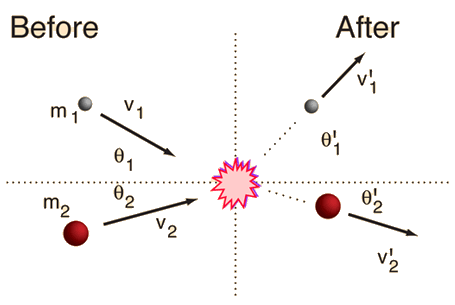# Collisions in Two Dimensions

In the general case of a two-dimensional collision between two masses, one cannot anticipate how much kinetic energy will be lost in the collision. Therefore, the velocities of the two masses after the collision are not completely determined by their velocities and directions before the collision. However, conservation of momentum must be satisfied, so that if the velocity of one of the particles after the collision is specified, the other is determined.
 Initial conditions: m1 =kg, v1 =m/s, θ1 =° m2 =kg, v2 =m/s, θ2 =°, KEi =JSince the momentum must be conserved in both magnitude and direction, the initial vector momentum must be found. This is done by resolving the momenta into components, using unit vector notation. The components are combined to give the total momentum of the system before the collision.
 p1 =i + j kg m/s p2 =i + j kg m/s pi =i + j kg m/s
 The initial momentum in polar form is pi =kg m/s at ° The angle here is the standard angle.
Enter values for masses and velocities above. Then specify one final velocity below.
 Final velocities(specify one, the other will be calculated): v'1 =m/s, θ'1 = °
 Final velocities(specify one, the other will be calculated): v'2 =m/s, θ'2 = °
 Final kinetic energy = KEf = J
If the calculation yields positive angles, that implies that they are in the sense given by the diagram. If the angles are negative, that means the angles swing the opposite direction from the x-axis than that indicated by the diagram.
For ordinary objects, the final kinetic energy will be less than the initial value. The only way you can get an increase in kinetic energy is if there is some kind of energy release triggered by the impact. Was one of your objects a hand grenade?

### Further discussion of concepts

Index

Collision concepts

Elastic collisions